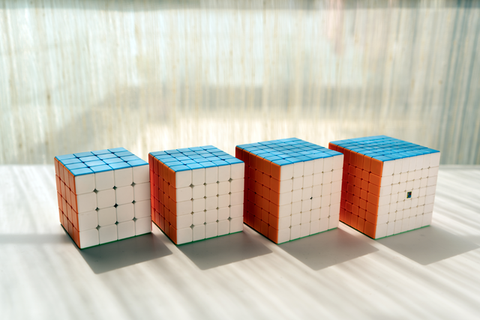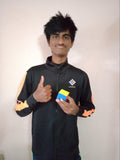## Introduction

While the Rubik’s Cube is fascinating just as a toy, improving at it requires one to learn the process of ‘scrambling’. In this process, we follow along with moves generated by a computer program, in order to obtain a state of the cube that is fully randomly generated. This is done either using websites such as cstimer.net or scramble-generating applications such as TNoodle (used by the WCA for official competitions).

## What Is Notation?

Notation refers to the combination of characters (such as alphabets) used to represent various turns on a puzzle, in the most intuitive way possible. So, it makes the process of scrambling uniform across the world, regardless of the cuber, software or cube being used.

Let us see what the basic idea behind notation is, first using the example of a 3x3 cube.

## The Logic

Taking any cubic puzzle, there are six faces, giving rise to 6 independently maneuverable layers. So, considering these, we have the Right, Left, Up, Down, Front and Back layers on every cube. To represent these, we make use of the alphabets R, L, U, D, F and B, respectively.

In order to determine the direction in which to move these layers, we make use of suffixes, like an apostrophe (read as “prime”) or 2 (for double turns). So, for example, R’ refers to a 90 degree counterclockwise rotation of the right layer. B2 refers to a 180 degree rotation of the back layer.

Some puzzles may have different scrambling techniques, such as the Clock, which has 9 dials and 2 faces, and involves a different notation (as will be discussed below). Similarly, even the Megaminx event has a different scrambling notation.

## Scrambling Orientation

The scrambles for most puzzles are done with green facing you and white on top. For the Pyraminx, we use green facing us and yellow on the bottom, while for the Skewb, we use a fixed-corner notation (FCN), with the red-green-white corner as a reference. For the Clock, we hold it with all the faces having dials with ‘12’ on top, while for the Square-1, we hold it with red facing us and yellow on top.

## The WCA Puzzles

For the 2x2 and 3x3 puzzles, the scrambling notation stays the same as discussed initially, with no changes/additions.

For 4x4 and 5x5, there is the inclusion of moves with the suffix “w”, implying that they are wide layer turns, or turns of two layers at a time. For example, Rw’ means both right layers are turned together, counterclockwise by 90 degrees.For 6x6 and 7x7, there is the inclusion of moves with a prefix “3”, in addition to the suffix “w”, which implies that you need to turn three layers at a time. For example, 3Lw2 means that all three of the left layers are turned together, by 180 degrees.

For the Pyraminx, the moves represent turns of the corresponding side of the tetrahedral shape (4 axes), according to the corners. Also, lowercase letters correspond to only moving the corner-most tips. So, B implies finding the corner at the back, and moving that segment of the puzzle clockwise. Similarly, r’ implies moving the right corner tip counterclockwise.

For the Skewb, since we have FCN (as described above), the moves imply moving the corresponding layers of the puzzle about that corner. As with the pyraminx, even the skewb has 4 axes, so, for example, R would imply a clockwise turn of that entire face about the fixed red-green-white corner.For the Megaminx, we scramble by treating the entire puzzle as segments, in such a way that R means every single layer except the single left-most layer. In addition, we have the suffixes “++” or “--” which show the direction (clockwise and counterclockwise, respectively) in which the corresponding part of the puzzle needs to be moved by that many (2) steps.

Only the R and D segments are used in the scrambling process, which means that the left and upper layers are used as fixed references and, for example, D++ means moving the entire lower section of the puzzle (except the single upper-most layer) clockwise by two steps (144 degrees).

For the Square-1, we have a completely unique system of scrambling, where the elements involved are the upper layer, lower layer and the transverse “slice” layer that runs across the puzzle almost vertically. A typical scramble has two numbers enclosed in parentheses separated by a comma. These are followed by a front slash “/”. Here, the first number implies how much to move the top layer while the second number implies how much to move the lower layer. The slash is a 180 degree motion of the full slice layer.

The sign convention used is that positive numbers mean clockwise and negative numbers mean counterclockwise. Every edge piece on each layer accounts for a value of ‘1’ and every corner piece a value of ‘2’. For example, (4,-3) implies that the upper layer is moved clockwise by an edge, a corner and another edge (1+2+1), while the lower layer is moved counterclockwise by an edge and a corner (1+2).For the Clock, it is yet another unique system, involving the pins on the faces and the gears to move the dials on the corners. The pins are all pushed down before the scramble, and the pins to be raised before making a certain move are implied by a pair of letters which show the location of the pin, or a single letter to show that both pins on that side of the clock need to be raised.

Then, the dial corresponding to that/those pin(s) is moved by a certain number of notches, again given by a number. If this number is positive, it’s clockwise and if negative, counterclockwise. The word ‘ALL’ is used to show that all pins need to be raised. In addition, ‘y2’ refers to flipping the clock over its side, and continuing the same process on the other side. At the end, certain moves are added to show the final position of the pins at the end of the scramble.

For example, DR3- implies that only the “Down-Right” pin should be raised and the corresponding dial is to be moved counterclockwise by 3 notches. U6+ implies that both upper pins are to be raised and any corresponding dial is to be moved clockwise by 6 notches.

1. What does ‘FRU’ mean on the Rubik’s Cube?

‘FRU’ refers to a sequence of moves, say within an algorithm, that only involve moving the Front, Right and Upper faces throughout. These are often referred to as ‘gen’. So, if I have an algorithm with only R and U turns, it is considered a ‘2-gen’ algorithm (RU-gen). Hence, an algorithm with only F, R and U turns is a ‘3-gen’ algorithm (FRU-gen).

2. What does the small ‘r’ denote on the Rubik’s Cube?

The small ‘r’ is also called the ‘fat r’ or ‘wide r’, and it refers to moving both of the right layers on the cube together. This makes notation easier, instead of having to write ‘L x’ (where x is a rotation), we can just write ‘r’. It is similar to writing ‘Rw’ for bigger cubes.

## Conclusion

This article shows the various intricacies and notations involved in scrambling every puzzle, and over time, one can get comfortable with all of these and have a smooth scrambling experience. Initially, it may seem tough, but with practice, it becomes second nature to us, and eventually we don’t even need to think about what we are doing as we scramble out of muscle memory!